# Linked list in C with example program

This post has been created and published to describe the implementation of linked lists in the C programming language. I already defined the "linked list" data structure in a separate post; therefore, in this post, I will only cover the implementation of it in C. However, let's start with its brief description.

In contrast to a stack or queue, a linked list allows for flexible access because each data item contains a link to the next data item in the chain. In addition, retrieving an item from a linked list does not remove or destroy it. To accomplish this, you must add a specialized deletion operation.

A linked list may be single- or double-linked. A singly linked list contains a link that points to the next data item. A doubly linked list, on the other hand, contains links to both the next and previous elements in the list. Depending on your application, you will employ one of these linked list types. Let's now examine singly linked lists.

## Singly Linked Lists in C

Each item in a singly-linked list must include a link to the following item. Each data item is typically comprised of a structure containing the data fields and a link pointer. Consider the following illustration, which depicts a singly linked list.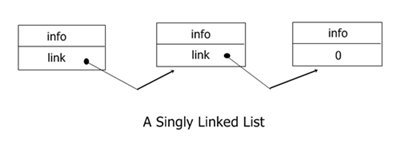Basically, there are two ways to build a singly linked list: The first is simply to put each new item at the end of the list. The other is to insert items into specific places in the list in ascending, sorted order.

The items stored in a linked list generally consist of structures because each item must carry with it a link to the next item in the list as well as the data itself. Hence, we will need to define a structure that will be used in the examples that follow. Since mailing lists are commonly stored in a linked list, an address structure is a good choice. The data structure for each element in the mailing list is defined here:

```struct address
{
char name;
char street;
char city;
char state;
char zip;
} info;```

The function slstore() shown here builds a singly linked list by placing each new element at the end. And it must be passed a pointer to a structure of type address that contains the new entry and a pointer to the last element in the list. In this case, if the list is empty, then the pointer to the last element in the list must be null. Here is the code fragment for the slstore() function:

```void slstore(struct address *i, struct address **last)
{
if(!*last) *last = i;    // first item in the list
else (*last)->next = i;
i->next = NULL;
*last = i;
}```

## Doubly Linked Lists in C

The doubly linked lists contain data as well as links to the next and previous items. Consider the following illustration, which depicts a doubly linked list.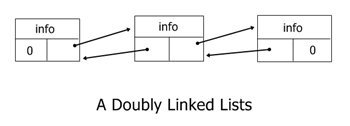Having two links as opposed to one has numerous benefits. Possibly the most crucial aspect is that the list can be read in either direction. This greatly simplifies list management by facilitating insertions and deletions. Additionally, we are able to scan the list in either direction. Another advantage is only relevant in the event of specific types of failure. Since the entire list can be read using either forward links or backward links, the list could be reconstructed using the other should one of the links become invalid.

There are three ways to insert a new element into a doubly-linked list:

• Insert a new first element.
• Insert in the middle.
• Insert a new last element.

Building a doubly linked list is similar to building a singly linked list, except that two links are maintained. Hence, the structure must have room for both links. Using the mailing list example again, you can modify the structure "address" as shown here to accommodate both links. Here is the code fragment:

```struct address
{
char name;
char street;
char city;
char state;
char zip;
} info;```

Using "address" as a basic data item, the following function, "dlstore(),"  builds a doubly linked list:

```void dlstore(struct address *i, struct address **last)
{
if(!*last)
*last = i;
else
(*last)->next = i;
i->next = NULL;
i->prior = *last;
*last = i;
}```

## Create a list in C

Let's create a program in C that creates the linked list based on the data entered by the user at program runtime.

```#include <stdlib.h>

struct node
{
int info;
struct node *next;
};
struct node *start = NULL;

int main()
{
struct node *temp, *ptr;
temp = (struct node *)malloc(sizeof(struct node));
if (temp == NULL)
{
printf("\nOut of Memory Space.\n");
return 0;
}
printf("Enter the data value for the node: ");
scanf("%d", &temp->info);
temp->next = NULL;
if (start == NULL)
start = temp;
else
{
ptr = start;
while (ptr->next != NULL)
ptr = ptr->next;
ptr->next = temp;
}
printf("\n");

return 0;
}```

## Create and display a list in C

Now is the time to add another feature to the program that allows the user to display the list created by him or her during the program's runtime.

```#include <stdlib.h>

void create();
void display();

struct node
{
int info;
struct node *next;
};

struct node *start = NULL;

int main()
{
int choice;
while (1)
{
printf("1. Create \n");
printf("2. Display \n");
printf("3. Exit \n\n");
scanf("%d", &choice);
switch (choice)
{
case 1:
create();
break;
case 2:
display();
break;
case 3:
return 0;

default:
printf("\nWrong Choice.\n\n");
break;
}
}
return 0;
}
void create()
{
struct node *temp, *ptr;
temp = (struct node *)malloc(sizeof(struct node));
if (temp == NULL)
{
printf("\nOut of Memory Space.\n");
exit(0);
}
printf("\nEnter the data value for the node: ");
scanf("%d", &temp->info);
temp->next = NULL;
if (start == NULL)
start = temp;
else
{
ptr = start;
while (ptr->next != NULL)
ptr = ptr->next;
ptr->next = temp;
}
printf("\nData inserted into the list.\n");
printf("\n");
}
void display()
{
struct node *ptr;
if (start == NULL)
{
printf("\nList is empty.\n");
return;
}
else
{
ptr = start;
printf("\nThe List elements are: ");
while (ptr != NULL)
{
printf("%d    ", ptr->info);
ptr = ptr->next;
}
}
printf("\n\n");
}```

The following snapshot shows the initial output produced by the above program: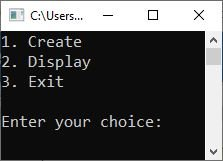Now type 1 and hit the ENTER key to get the following output: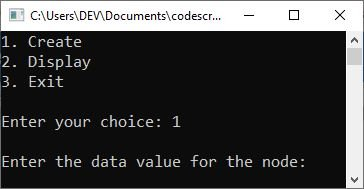Enter the data for the node, say 10, and hit the ENTER key to get the following output: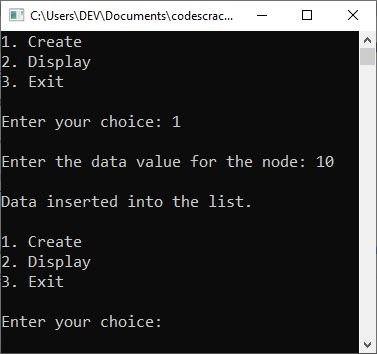Let me try the first option again to enter another node with data value 20, then the second option to display the list, and the third option to exit the program.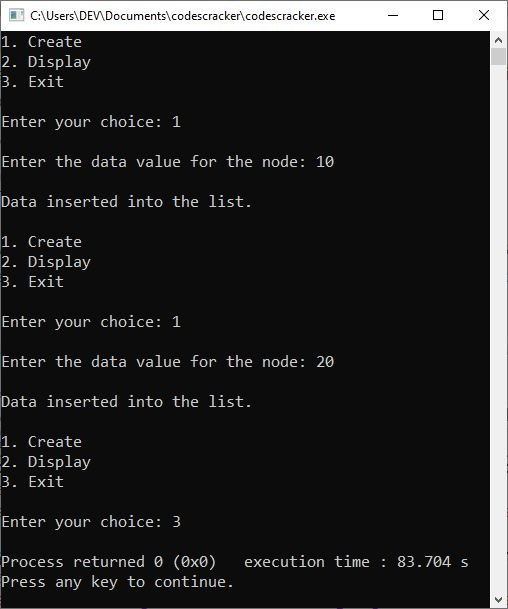Following is a list of additional features you can add to the previous program:

Add a new feature to the option using the previous program. Include the corresponding code in the function definition to provide another feature to the user. That is, define the function's prototype in the same way that you did for "creating" and "displaying" the list, add another option, and then create another case to call the function associated with it. Use the corresponding code from these six features or sections in the function definition.

## Insert at the beginning of a list in C

```struct node *temp;
temp = (struct node *)malloc(sizeof(struct node));
if (temp == NULL)
{
printf("\nOut of Memory Space.\n");
return;
}
printf("\nEnter the data value for the node: ");
scanf("%d", &temp->info);
temp->next = NULL;
if (start == NULL)
{
start = temp;
}
else
{
temp->next = start;
start = temp;
}
printf("\n");```

## Insert at the end of a list in C

```struct node *temp, *ptr;
temp = (struct node *)malloc(sizeof(struct node));
if (temp == NULL)
{
printf("\nOut of Memory Space.\n");
return;
}
printf("\nEnter the data value for the node: ");
scanf("%d", &temp->info);
temp->next = NULL;
if (start == NULL)
{
start = temp;
}
else
{
ptr = start;
while (ptr->next != NULL)
{
ptr = ptr->next;
}
ptr->next = temp;
}
printf("\n");```

## Insert at the specified position in a list in C

```struct node *ptr, *temp;
int i, pos;
temp = (struct node *)malloc(sizeof(struct node));
if (temp == NULL)
{
printf("\nOut of Memory Space.\n");
return;
}
printf("\nEnter the position for the new node to be inserted: ");
scanf("%d", &pos);
printf("\nEnter the data value of the node: ");
scanf("%d", &temp->info);

temp->next = NULL;
if (pos == 0)
{
temp->next = start;
start = temp;
}
else
{
for (i = 0, ptr = start; i < pos - 1; i++)
{
ptr = ptr->next;
if (ptr == NULL)
{
return;
}
}
temp->next = ptr->next;
ptr->next = temp;
}
printf("\n");```

## Delete from the beginning of a list in C

```struct node *ptr;
if (ptr == NULL)
{
printf("\nList is Empty.\n");
return;
}
else
{
ptr = start;
start = start->next;
printf("\nThe deleted element is: %d \n", ptr->info);
free(ptr);
}
printf("\n");```

## Delete from the end of a list in C

```struct node *temp, *ptr;
if (start == NULL)
{
printf("\nList is Empty.\n");
exit(0);
}
else if (start->next == NULL)
{
ptr = start;
start = NULL;
printf("\nThe deleted element is: %d \n", ptr->info);
free(ptr);
}
else
{
ptr = start;
while (ptr->next != NULL)
{
temp = ptr;
ptr = ptr->next;
}
temp->next = NULL;
printf("\nThe deleted element is: %d \n", ptr->info);
free(ptr);
}
printf("\n");```

## Delete from the specified position in a list in C

```int i, pos;
struct node *temp, *ptr;
if (start == NULL)
{
printf("\nThe List is Empty.\n");
exit(0);
}
else
{
printf("\nEnter the position of the node to be deleted: ");
scanf("%d", &pos);
if (pos == 0)
{
ptr = start;
start = start->next;
printf("\nThe deleted element is: %d \n", ptr->info);
free(ptr);
}
else
{
ptr = start;
for (i = 0; i < pos; i++)
{
temp = ptr;
ptr = ptr->next;
if (ptr == NULL)
{
return;
}
}
temp->next = ptr->next;
printf("\nThe deleted element is: %d \n", ptr->info);
free(ptr);
}
}
printf("\n");```

C Online Test

« Previous Tutorial Next Tutorial »